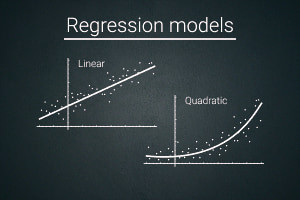Data Analytics | Regression Models | Free Online Course | Alison# Data Analytics: Regression Models

## This free online takes you through the details the techniques and frameworks used in regression modelling and analysis

Data Science
Free Course
Regression analysis is used to model the relationship between a response variable and one or more predictor variables. Estimation is a key factor used in regression analysis. This free online course focuses on maximum likelihood estimation as a probabilistic framework for predicting the probability distribution and parameters that describe observed data. By the end of this course, you will become familiar with the techniques used in regression.

3-4 Hours

Yes

Yes

Yes
• ### Publisher

NPTEL## Description

Regression models analysis is a predictive modelling technique used for determining the relationship between dependent and independent variables. This free online course on Alison describes the point and interval estimation as well as the differences between these estimations, including how point estimations uses a single value while interval estimation uses a range of numbers to get information from the population. You will be introduced to the different types of residual analysis.

This course describes how the Python code is used for the scatter plot diagram and for the regression equation. You will learn about the importance of assumptions on error terms. You will also learn about the concept of the assumed regression model and the differences between simple and multiple regression. You will also be introduced to the assumed regression model and how it is not an adequate representation.

Lastly, this course explains the intuition behind the maximum likelihood estimation theory. You will learn about the estimation of Poisson and exponential distribution parameters. You will study the python demo for the estimation of population parameters for the regression equation. This course also takes you through the relationship between the odds ratio and the coefficients of independent variables. If you are interested in learning how regression analysis models are used for forecasting, then this exciting course is for you.

Start Course Now

## Learning Outcomes

Upon successful completion of this course, you will be able to:

• Discuss the intuition behind the maximum likelihood estimation theory
• Explain the graph of the natural logarithm of the likelihood
• Describe the estimation of Poisson and exponential distribution parameter
• Discuss the python demo for estimation of population parameters for regression equation
• Explain the graphical illustration of likelihood contribution
• Describe how to build the logistic regression model and equation
• Discuss the intuition behind the maximum likelihood estimation theory
• Explain the graph of the natural logarithm of the likelihood
• Describe the estimation of Poisson and exponential distribution parameter
• Discuss the python demo for estimation of population parameters for regression equation
• Describe the relationship between the odds ratio and the coefficients of independent variables

## Certification

All Alison courses are free to enrol, study and complete. To successfully complete this Certificate course and become an Alison Graduate, you need to achieve 80% or higher in each course assessment. Once you have completed this Certificate course, you have the option to acquire an official Certificate, which is a great way to share your achievement with the world. Your Alison Certificate is:

Ideal for sharing with potential employers - include it in your CV, professional social media profiles and job applications
An indication of your commitment to continuously learn, upskill and achieve high results
An incentive for you to continue empowering yourself through lifelong learning

Alison offers 3 types of Certificates for completed Certificate courses:

Digital Certificate - a downloadable Certificate in PDF format, immediately available to you when you complete your purchase
Certificate - a physical version of your officially branded and security-marked Certificate, posted to you with FREE shipping
Framed Certificate - a physical version of your officially branded and security-marked Certificate in a stylish frame, posted to you with FREE shipping